# The periodicity of the function

Definition: a Functionis called periodic with period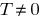if for anyof the identifying numbers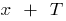and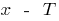also included in the scope and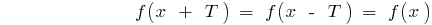.

## Properties of periodic functions

1. If the number is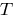a period function, then the number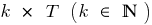is also the period of the function.
2. If the function is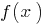periodic with a period, the function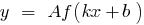is also periodic and its period is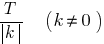3. If the function is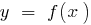periodic with period, then the composed function (function of function)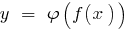is also periodic with period.
4. To plot periodic functions with the period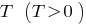enough to graph on the interval length, and then in parallel to shift this graph along the axis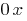at a distance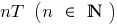left and right.

## Examples of periodic functions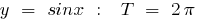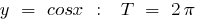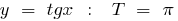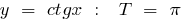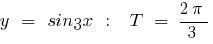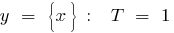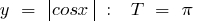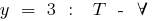Tags:
Chapter:
Versions in other languages:
Share with friends: